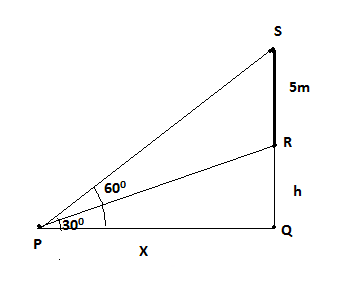Courses
Courses for Kids
Free study material
Free LIVE classes
MoreLIVE
Join Vedantu’s FREE Mastercalss

# A vertical tower stands on a horizontal plane and is surmounted by a vertical flagstaff of height 5 meters. At a point on the plane, the angles of elevation of the bottom and the top of the flagstaff are respectively ${30^0}$ and ${60^0}$.Find the height of the tower.Verified
265.9k+ views
Hint- Draw the diagram of question and use value of trigonometric angles $\tan {30^0} = \frac{1}{{\sqrt 3 }}$ and $\tan {60^0} = \sqrt 3$ .

Let QR be the height of the tower (h meters) and RS be the height of flagstaff surmounted on
the tower (RS=5m). Let the point P lie on the horizontal plane at a distance of x meters from
the foot of the tower at point Q (PQ=x meters).In $\vartriangle PQR$, the angle of elevation of the bottom of the flagstaff is ${30^0}$ .
$\tan {30^0} = \dfrac{{QR}}{{PQ}} \\ \Rightarrow \dfrac{1}{{\sqrt 3 }} = \frac{h}{x} \\ \Rightarrow x = \sqrt 3 h.........\left( 1 \right) \\$
In $\vartriangle PQS$, angle of elevation of the top of the flagstaff is ${60^0}$ .
$\tan {60^0} = \dfrac{{QS}}{{PQ}} = \dfrac{{QR + RS}}{{PQ}} \\ \Rightarrow \sqrt 3 = \dfrac{{h + 5}}{x} \\ \Rightarrow x = \dfrac{{h + 5}}{{\sqrt 3 }}..........\left( 2 \right) \\$
Eliminating x using (1) and (2) equation
$\Rightarrow \sqrt 3 h = \dfrac{{h + 5}}{{\sqrt 3 }} \\ \Rightarrow 3h = h + 5 \\ \Rightarrow 2h = 5 \\ \Rightarrow h = 2.5m \\$
So, the height of tower is 2.5 meters

Note- Whenever we face such types of problems we use some important points. Like draw
the figure of question with notify all points and distances then make the relation between
variables with the help of trigonometric angles then after eliminating x we can get the value
of the height of the tower.

Last updated date: 29th Sep 2023
Total views: 265.9k
Views today: 3.65k